# Effectiveness of classification for CART® Classification

Select the method or formula of your choice.

These statistics appear in the table that shows the effectiveness of the classification for the best and worst terminal nodes. In general, rows are in order by the size of the class probabilities. Values within 1E-12 are ties. Minitab sorts ties by their weighted counts. If the weighted counts are also ties, then Minitab sorts ties by the node ID.

## % of N

The following formula gives the percent of the data that are in the node. This formula is for data without weights:

The following formula is for data with weights:

### Notation

TermDescription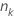number of cases in node k
Nnumber of cases in the data set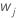weight for the jth case in the node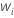weight for the ith case in the data set

## Event prob and non-event prob

Minitab refers to event probability and non-event probability for binary response variables.
The following formula gives the percent events in the node. This formula is for data without weights:

The following formula is for data with weights:

The non-event probability has the following definition:

### Notation

TermDescription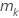number of events in node knumber of cases in node kweight for the jth event in the nodeweight for the ith observation in the node

## Class prob and non-class prob

Minitab refers to class probability and non-class probability for multinomial response variables.
The following formula gives the percent for a class in the node. This formula is for data without weights.

The following formula is for data with weights:

The non-class probability has the following definition:

### Notation

TermDescription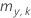number of cases in a class y in node knumber of cases in node kweight for the jth case in class y in the nodeweight for the ith case in the node

## Odds

The formula for odds depends on whether the response variable is binary or multinomial.

### Multinomial response variable

By using this site you agree to the use of cookies for analytics and personalized content.  Read our policy